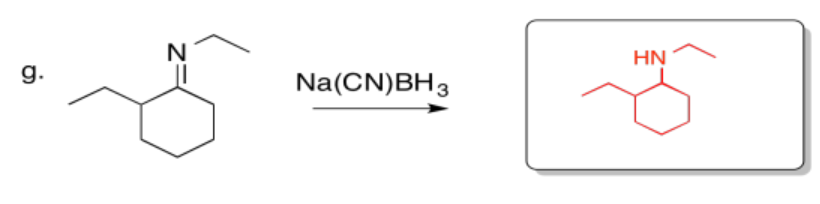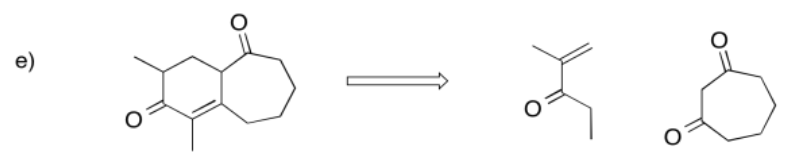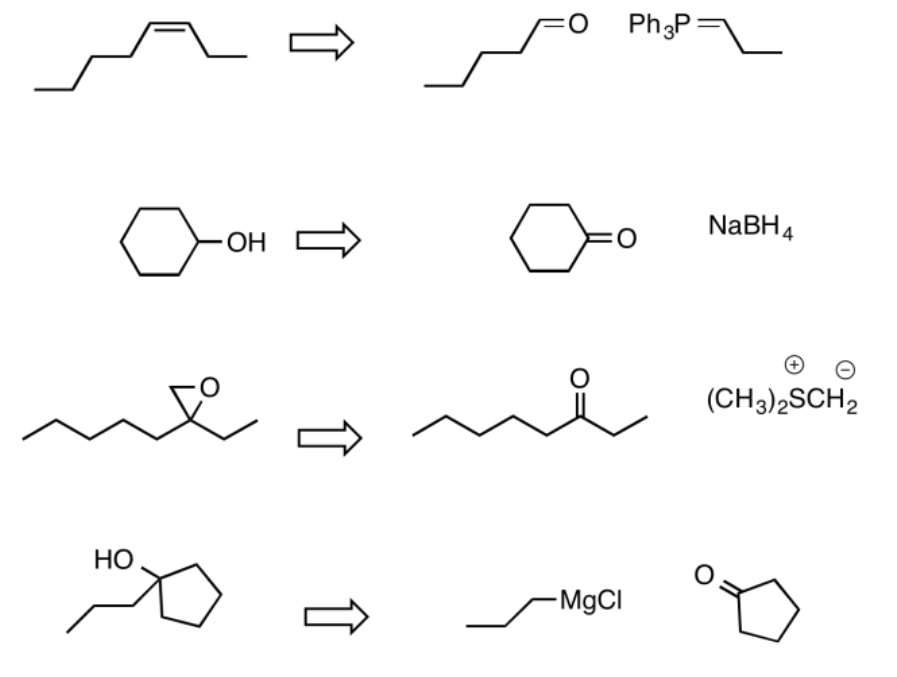# 3.20: Solutions to Selected Problems, Part BFigure $$\PageIndex{1}$$:Figure $$\PageIndex{2}$$:Figure $$\PageIndex{3}$$:Figure $$\PageIndex{4}$$:Figure $$\PageIndex{5}$$:

Part a.Figure $$\PageIndex{6}$$:

Part b.Figure $$\PageIndex{7}$$:Figure $$\PageIndex{8}$$:Figure $$\PageIndex{9}$$:Figure $$\PageIndex{10}$$:Figure $$\PageIndex{11}$$:Figure $$\PageIndex{12}$$:Figure $$\PageIndex{13}$$:Figure $$\PageIndex{14}$$:Figure $$\PageIndex{15}$$:Figure $$\PageIndex{16}$$:Figure $$\PageIndex{17}$$:Figure $$\PageIndex{18}$$:Figure $$\PageIndex{19}$$:Figure $$\PageIndex{20}$$:Figure $$\PageIndex{21}$$:Figure $$\PageIndex{22}$$:Figure $$\PageIndex{23}$$:Figure $$\PageIndex{24}$$:Figure $$\PageIndex{25}$$:Figure $$\PageIndex{26}$$:Figure $$\PageIndex{27}$$:Figure $$\PageIndex{28}$$:Figure $$\PageIndex{29}$$:Figure $$\PageIndex{30}$$:Figure $$\PageIndex{31}$$:Figure $$\PageIndex{32}$$:Figure $$\PageIndex{33}$$:Figure $$\PageIndex{34}$$:Figure $$\PageIndex{35}$$:

One of the keys in this problem is recognizing that in some steps, two different reactions are involved. For example, in the first box, there is an addition of a diol to a carbonyl followed by an ylide addition.Figure $$\PageIndex{36}$$:Figure $$\PageIndex{37}$$:Figure $$\PageIndex{38}$$:Figure $$\PageIndex{39}$$:Figure $$\PageIndex{40}$$:Figure $$\PageIndex{41}$$:

a)Figure $$\PageIndex{42}$$:

b) The circled part changed from a single bond to a doube bond. The boxed part changed from an alcohol to unadorned hydrocarbon chain.

c) Hydrogen bonding is the most obvious.

d) However, the analog works better without this group; this particular alcohol group is probably not an important part of the pharmacophore. It is probably not needed in order to bind to the target.

e) In epothilone A, as drawn, the dihedral angle appears to be 0 degrees.

f) In the new analog, the dihedral angle is 180 degrees.

g) Based on the superior activity of the analog, the active conformation of the ring is probably more like the one on the right than the one on the left. The circled bond probably adopts a dihedral angle closer to 180 degrees, with the rest of the ring twisting into a shape more like the one shown on the right, in order to bind to the target.Figure $$\PageIndex{43}$$:Figure $$\PageIndex{44}$$:Figure $$\PageIndex{45}$$:Figure $$\PageIndex{46}$$:Figure $$\PageIndex{47}$$:Figure $$\PageIndex{48}$$:Figure $$\PageIndex{49}$$:

a)Figure $$\PageIndex{50}$$:Figure $$\PageIndex{51}$$:Figure $$\PageIndex{52}$$:Figure $$\PageIndex{53}$$:Figure $$\PageIndex{54}$$:Figure $$\PageIndex{55}$$:Figure $$\PageIndex{56}$$:Figure $$\PageIndex{57}$$:Figure $$\PageIndex{58}$$:Figure $$\PageIndex{59}$$:Figure $$\PageIndex{60}$$:Figure $$\PageIndex{61}$$:Figure $$\PageIndex{62}$$:Figure $$\PageIndex{63}$$:Figure $$\PageIndex{64}$$:Figure $$\PageIndex{65}$$:Figure $$\PageIndex{66}$$:Figure $$\PageIndex{67}$$:Figure $$\PageIndex{68}$$:Figure $$\PageIndex{69}$$:Figure $$\PageIndex{70}$$:Figure $$\PageIndex{71}$$:Figure $$\PageIndex{72}$$:Figure $$\PageIndex{73}$$:Figure $$\PageIndex{74}$$:Figure $$\PageIndex{75}$$:Figure $$\PageIndex{76}$$:Figure $$\PageIndex{77}$$:Figure $$\PageIndex{78}$$:Figure $$\PageIndex{79}$$:Figure $$\PageIndex{80}$$:Figure $$\PageIndex{81}$$:Figure $$\PageIndex{82}$$:Figure $$\PageIndex{83}$$:

Problem CO26.8.Figure $$\PageIndex{84}$$:Figure $$\PageIndex{85}$$:Figure $$\PageIndex{86}$$: